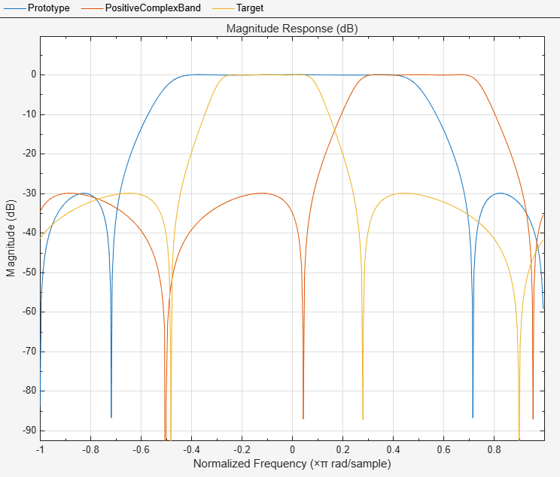# iirbpc2bpc

Transform IIR complex bandpass filter to IIR complex bandpass filter with different characteristics

## Syntax

``````[Num,Den,AllpassNum,AllpassDen] = iirbpc2bpc(B,A,Wo,Wt)``````

## Description

example

``````[Num,Den,AllpassNum,AllpassDen] = iirbpc2bpc(B,A,Wo,Wt)``` transform IIR complex bandpass filter to IIR complex bandpass filter with different characteristics.The `iirbpc2bpc` function returns the numerator and denominator vectors, `Num` and `Den`, respectively of the target filter transformed from the complex bandpass prototype by applying a first-order complex bandpass to complex bandpass frequency transformation. For more details, see IIR Complex Bandpass Frequency Transformation.The function also returns the numerator, `AllpassNum`, and the denominator, `AllpassDen`, of the allpass mapping filter. The prototype lowpass filter is specified with the numerator `B` and a denominator `A`.Frequencies must be normalized to be between -1 and 1, with 1 corresponding to half the sample rate.```

## Examples

collapse all

Design a prototype real IIR lowpass elliptic filter with a gain of about –3 dB at 0.5π rad/sample.

`[b,a] = ellip(3,0.1,30,0.409);`

Create a complex passband from 0.25π to 0.75π.

`[bc,ac] = iirlp2bpc(b,a,0.5,[0.25 0.75]);`

Move the bandpass to between –0.3π and 0.1π.

`[num,den] = iirbpc2bpc(bc,ac,[0.25 0.75],[-0.3 0.1]);`

Compare the three filters in FVTool.

```hvft = fvtool(b,a,bc,ac,num,den); legend(hvft,'Prototype','Positive complex band','Target')```## Input Arguments

collapse all

Numerator of the prototype lowpass filter, specified as a row vector.

Data Types: `single` | `double`
Complex Number Support: Yes

Denominator of the prototype lowpass filter, specified as a row vector.

Data Types: `single` | `double`
Complex Number Support: Yes

Frequency values to be transformed from the prototype filter, specified as a two-element vector. Frequencies in `Wo` must be normalized to be between -1 and 1, with 1 corresponding to half the sample rate.

Data Types: `single` | `double`

Desired frequency locations in the transformed target filter, specified as a two-element vector. Frequencies in `Wt` must be normalized to be between -1 and 1, with 1 corresponding to half the sample rate.

Data Types: `single` | `double`

## Output Arguments

collapse all

Numerator of the target filter, returned as a row vector.

Data Types: `single` | `double`
Complex Number Support: Yes

Denominator of the target filter, returned as a row vector.

Data Types: `single` | `double`
Complex Number Support: Yes

Numerator of the mapping filter, returned as a row vector.

Data Types: `single` | `double`
Complex Number Support: Yes

Denominator of the mapping filter, returned as a row vector.

Data Types: `single` | `double`

collapse all

### IIR Complex Bandpass Frequency Transformation

IIR Complex Bandpass Frequency transformation effectively places two features of the original filter, located at frequencies Wo1 and Wo2, at the required target frequency locations, Wt1, and Wt2 respectively. It is assumed that Wt2 is greater than Wt1. In most of the cases the features selected for the transformation are the band edges of the filter passbands. In general it is possible to select any feature; e.g., the stopband edge, the DC, the deep minimum in the stopband, or other ones.

Relative positions of other features of the original filter do not change in the target filter. This means that it is possible to select two features of an original filter, F1 and F2, with F1 preceding F2. Feature F1 will still precede F2 after the transformation. However, the distance between F1 and F2 will not be the same before and after the transformation.

IIR complex bandpass frequency transformation can also be used for transforming other types of filters; e.g., complex notch filters or resonators can be repositioned at two distinct desired frequencies at any place around the unit circle for example in the adaptive system.

## Version History

Introduced in R2011a